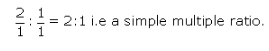# What is the law of reciprocal proportion?

what is the law of reciprocal proportion?

This law was proposed by Ritcher in 1792. It states that ‘when two different elements combine separately with the same weight of a third element, the ratio of the masses in which they do so will be the same or some simple multiple of the mass ratio in which they combine with each other’.
For example, oxygen and sulphur react with copper to give copper oxide and copper sulphide respectively. Suphur and oxygen also react with each other to give SO2.
In CuS, Cu:S = 63.5:32
In CuO, Cu:O = 63.5:16
S:O = 32:16
S:O = 2:1
Now in SO S:O = 32:32
S:O = 1:1
Thus the ratio between the two ratios is